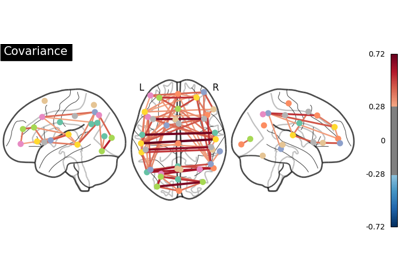Note

This page is a reference documentation. It only explains the class signature, and not how to use it. Please refer to the user guide for the big picture.

# nilearn.connectome.GroupSparseCovarianceCV#

class nilearn.connectome.GroupSparseCovarianceCV(alphas=4, n_refinements=4, cv=None, tol_cv=0.01, max_iter_cv=50, tol=0.001, max_iter=100, verbose=0, n_jobs=1, debug=False, early_stopping=True)[source]#

Sparse inverse covariance w/ cross-validated choice of the parameter.

A cross-validated value for the regularization parameter is first determined using several calls to group_sparse_covariance. Then a final optimization is run to get a value for the precision matrices, using the selected value of the parameter. Different values of tolerance and of maximum iteration number can be used in these two phases (see the tol and tol_cv keyword below for example).

Parameters:
alphasinteger, optional

initial number of points in the grid of regularization parameter values. Each step of grid refinement adds that many points as well. Default=4.

n_refinementsinteger, optional

number of times the initial grid should be refined. Default=4.

cvinteger, optional

number of folds in a K-fold cross-validation scheme. If None is passed, defaults to 3.

tol_cvfloat, optional

tolerance used to get the optimal alpha value. It has the same meaning as the tol parameter in `group_sparse_covariance`. Default=1e-2.

max_iter_cvinteger, optional

maximum number of iterations for each optimization, during the alpha- selection phase. Default=50.

tolfloat, optional

tolerance used during the final optimization for determining precision matrices value. Default=1e-3.

max_iterinteger, optional

maximum number of iterations in the final optimization. Default=100.

verboseinteger, optional

verbosity level. 0 means nothing is printed to the user. Default=0.

n_jobsinteger, optional

maximum number of cpu cores to use. The number of cores actually used at the same time cannot exceed the number of folds in folding strategy (that is, the value of cv). Default=1.

debugbool, optional

if True, activates some internal checks for consistency. Only useful for nilearn developers, not users. Default=False.

early_stoppingbool, optional

if True, reduce computation time by using a heuristic to reduce the number of iterations required to get the optimal value for alpha. Be aware that this can lead to slightly different values for the optimal alpha compared to early_stopping=False. Default=True.

Notes

The search for the optimal penalization parameter (alpha) is done on an iteratively refined grid: first the cross-validated scores on a grid are computed, then a new refined grid is centered around the maximum, and so on.

Attributes:
`covariances_`numpy.ndarray, shape (n_features, n_features, n_subjects)

covariance matrices, one per subject.

`precisions_`numpy.ndarray, shape (n_features, n_features, n_subjects)

precision matrices, one per subject. All matrices have the same sparsity pattern (if a coefficient is zero for a given matrix, it is also zero for every other.)

`alpha_`float

penalization parameter value selected.

`cv_alphas_`list of floats

all values of the penalization parameter explored.

`cv_scores_`numpy.ndarray, shape (n_alphas, n_folds)

scores obtained on test set for each value of the penalization parameter explored.

__init__(alphas=4, n_refinements=4, cv=None, tol_cv=0.01, max_iter_cv=50, tol=0.001, max_iter=100, verbose=0, n_jobs=1, debug=False, early_stopping=True)[source]#
fit(subjects, y=None)[source]#

Compute cross-validated group-sparse precisions.

Parameters:
subjectslist of numpy.ndarray with shapes (n_samples, n_features)

input subjects. Each subject is a 2D array, whose columns contain signals. Sample number can vary from subject to subject, but all subjects must have the same number of features (i.e. of columns.)

Returns:
selfGroupSparseCovarianceCV

the object instance itself.

get_params(deep=True)#

Get parameters for this estimator.

Parameters:
deepbool, default=True

If True, will return the parameters for this estimator and contained subobjects that are estimators.

Returns:
paramsdict

Parameter names mapped to their values.

set_params(**params)#

Set the parameters of this estimator.

The method works on simple estimators as well as on nested objects (such as `Pipeline`). The latter have parameters of the form `<component>__<parameter>` so that it’s possible to update each component of a nested object.

Parameters:
**paramsdict

Estimator parameters.

Returns:
selfestimator instance

Estimator instance.

## Examples using `nilearn.connectome.GroupSparseCovarianceCV`#Connectivity structure estimation on simulated data

Connectivity structure estimation on simulated dataGroup Sparse inverse covariance for multi-subject connectome

Group Sparse inverse covariance for multi-subject connectome